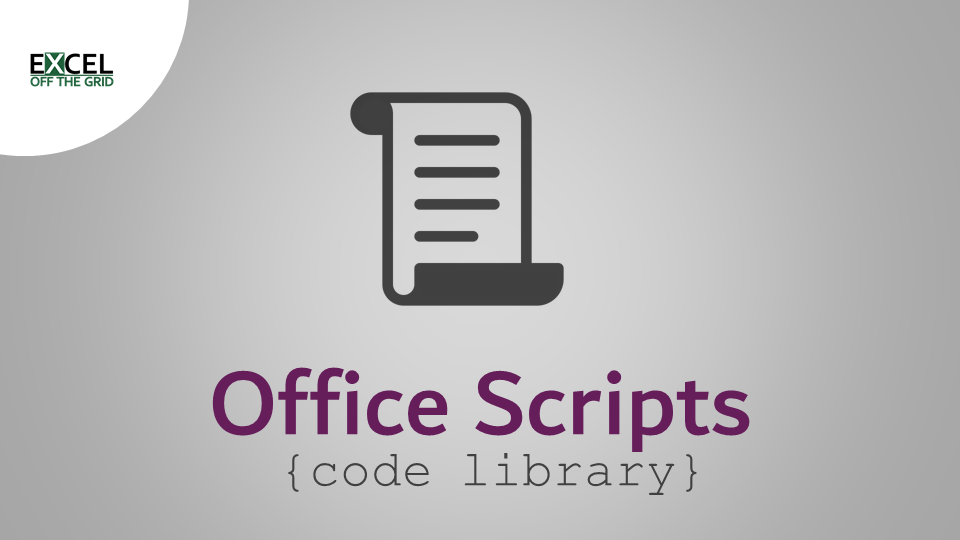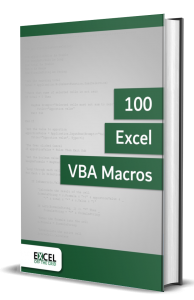# Office Scripts – Reverse number signsWe often need to reverse number signs in Excel.  For me, this regularly occurs because the accounting signage for debits and credits is opposite to the signage of income and costs.  I am sure in your chosen field there are plenty of scenarios also.  Using native Excel functionality, there are plenty of ways to change numbers from positive to negative or vice versa.

Those native Excel methods are all well and good, but it can become a bit tiresome if we have to do it a lot.  If we’re using Excel online, having an Office Script perform the task for us can be much faster.  So, in this post, we’ll cover the code necessary to achieve this.

Contents

## Technique

When using Office Scripts, the most common reason for slow running scripts is the amount of interaction with the workbook.  Therefore, it is optimal to read and write the data from and to the workbook as little as possible.  Anything which loops over a range cell by cell is likely to be very slow.  Instead, we can use arrays.  The high-level process is:

1. Read the data into an array
2. Manipulate the array
3. Push the result back to the workbook

When using arrays, we have two good options for manipulating each item:

1. for loop
2. map method (which is specific for arrays).

For the purposes of flipping number signs, both achieve similar results and could be used interchangeably.  Therefore, in this post, I have included both methods so you can choose whichever you prefer.

## Reverse number signs when all cells are values

If all the cells in a range contain values, the signage flipping can be applied to every item in the array.

The code examples in this section work with cell values; positive numbers are converted to negative, and negative are converted to positive.  As the script runs on values, it also converts any formulas to hardcoded values.

### for loop approach

```function main(workbook: ExcelScript.Workbook)
{

//Declare and assign variable for the selected cells
let rng = workbook.getSelectedRange();

//Create array for the values in the range
let arr = rng.getValues();

//Loop through each item in the 2D array
for (let i = 0; i < arr.length; i++) {
for (let j = 0; j < arr.length; j++) {

//Flip signage value
arr[i][j] = -arr[i][j]

}
}

//Push array back to cells using the setValues method
rng.setValues(arr);

};```

### Array map approach

```function main(workbook: ExcelScript.Workbook)
{

//Declare and assign variable for the selected cells
let rng = workbook.getSelectedRange();

//Create array for the values in the range
let arr = rng.getValues();

//Map through each item in the 2D array
let newArr = arr.map(row => {
return row.map (cell => {

//Flip signage value
return -cell;

})
});

//Push array back to cells using the setValues method
rng.setValues(newArr);

};```
100 Excel VBA Macros

Do you know the fastest way to learn foreign languages?  It is to read, write, speak, and think in that language as often as possible.  Apart from speaking, programming languages are no different.  The more you immerse yourself in that language, the faster you will pick it up.Therefore, what most people like you need is lots of examples that you can practice.  That is why the 100 Excel VBA Macros eBook exists.  It’s the book for all Excel users who want to learn how to read and write Excel macros, save time, and stand out from their peers.  The book contains:

• 100 example codes to practice reading and writing macros that will embed the language into your thinking.
• An introduction to macros in Excel to ensure you can implement the VBA code in the book even if you have no prior knowledge.
• Consistent code layout between examples to enable you to understand the structure and easily customize the code to meet your needs.
• Downloadable workbook containing all the source code, so the examples can be added to your project to give you the benefit of VBA straight away.

## Reverse number signs when cells are mixed types

In the code below, we tackle the issue of formulas or text within the selected data range.  The key differences are:

• Rather than the getValues and setValues methods, it uses the getFormulas and setFormulas.
• An additional check has been added to test if the array element contains a number.
• We use isNaN to assess if a value is not a number.  true = not a number, false = it is a number.
• ! before isNaN reverses the true/false result.
• The action to reverse number signs is only applied to those items that are numbers.

If the range contains any numbers preceded by an equals ( = ) symbol, these are treated as formulas.  For example:

• 12 is a cell value
• =12 is a formula in which the result is 12

### for loop approach

```function main(workbook: ExcelScript.Workbook)
{

//Declare and assign variable for the selected cells
let rng = workbook.getSelectedRange();

//Create array for the values in the range
let arr = rng.getFormulas();

//Loop through each item in the 2D array
for (let i = 0; i < arr.length; i++) {
for (let j = 0; j < arr.length; j++) {

//Check if the array item is a number
if (!isNaN(arr[i][j])) {

//Flip signage value
arr[i][j] = -arr[i][j]

}
}
}

//Push array back to cells using the setFormulas method
rng.setFormulas(arr);

};
```

### Array map method

```function main(workbook: ExcelScript.Workbook)
{

//Declare and assign variable for the selected cells
let rng = workbook.getSelectedRange();

//Create array for the values in the range
let arr = rng.getFormulas();

//Map through each item in the 2D array
let newArr = arr.map(row => {
return row.map (cell => {

//Check item is a number, if true reverse
if (!isNaN(cell)) {
return -cell;
} else {
return cell;
}
})
});

//Push array back to cells using the setFormulas method
rng.setFormulas(newArr);

};```Get our FREE VBA eBook of the 30 most useful Excel VBA macros.
Automate Excel so that you can save time and stop doing the jobs a trained monkey could do.

Email Address * First Name *

Don’t forget: